Sie sind hier: ICP » R. Hilfer » Publikationen

# 1 Introduction

[page 1, §1]
[1.1.1] Given the connection (established in [1, 2]) between continuous time random walks (CTRW) and diffusion equations with fractional time derivative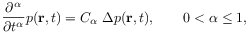(1)

it has been subsequently argued in the literature that all continuous time random walks with long tailed waiting time densities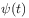, i.e. with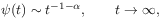(2)

are in some sense asymptotically equivalent to a fractional diffusion equation [3, 4, 5, 6, 7, 8]. [1.1.2] Let me first explain the symbols in these two equations. [1.1.3] Of course the fractional time derivative of order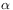in (1) is only a symbolic notation (a [page 2, §0]   definition is given in eq. (13) below). [2.0.1] Random walks on a lattice in continuous time are described by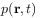, the probability density to find a random walker at the (discrete) lattice position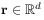at time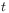if it started from the origin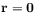at time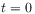[9, 10]. [2.0.2] In eq. (2) the waiting time distributiongives the probability density for a time intervalbetween two consecutive steps of the random walker, and the long time tail exponentis the same as the order of the fractional time derivative in (1) (see [1, 2] for details). [2.0.3] As usual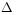denotes the Laplacian and the constant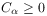denotes the fractional diffusion coefficient.

[2.1.1] Despite early doubts, formulated e.g. in [11, p. 78], many authors [3, 4, 5, 6, 7, 8] consider it now an established fact that proposition Asatisfies a fractional diffusion equation” and proposition Bis the solution of a CTRW with long time tail” are in some sense asymptotically equivalent. [2.1.2] Equivalence between propositions A and B requires that A implies B and further that B implies A. [2.1.3] One implication, namely that A implies B, was shown to be false in Refs.  and [13, p. 116ff] by showing that fractional diffusion equations of orderand type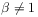(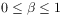) do not have a probabilistic interpretation.

[2.2.1] In this paper an example of a CTRW is given whose waiting time density fulfills eq. (2) but whose asymptotic continuum limit is not the fractional diffusion equation (1) (with the same). [2.2.2] Naturally, the idea underlying the example can be widely generalized.